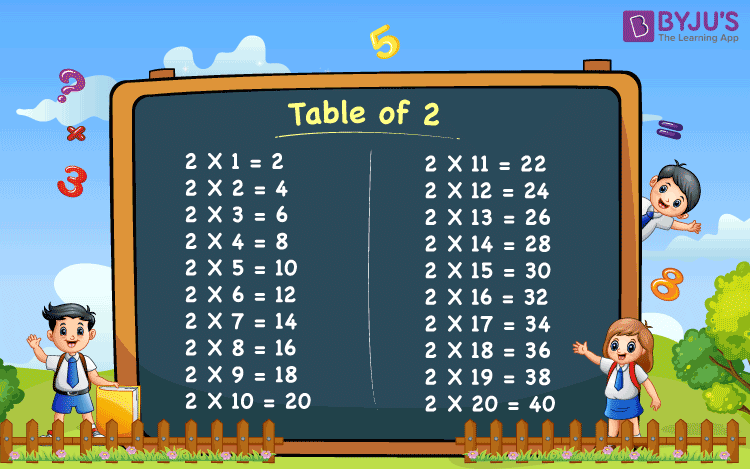# Table of 2

Table of 2 is the 2 times multiplication table that includes the multiples of number 2. 2 times table represents the repeated addition of whole number 2 when multiplied by any number. For example, when 2 is multiplied by 3, then 2 is added to itself, three times, such that 2 x 3 = 2+2+2 = 6. Hence, when 2 is multiplied by different numbers, then the resulting values are different. We can get table 2 by multiplying it with consecutive positive integers.

Table of 2 is provided below so that students can do the fast calculation. This helps them to save a lot of time in the time-based competitive examination. We have provided here the multiplication table of 2 up to 20 times in different formats to help students in memorising this table.

## Table of 2 Chart

Kids learn quickly by visual representation or charts. Below is the chart of the table of 2, to make children learn fast.## Multiplication Table of 2

Table of 2 is the most fundamental table every student should learn. It forms the base of multiplication problems. Below is the table of 2 given up to 20 times.

 2 x 1 = 2 2 x 2 = 4 2 x 3 = 6 2 x 4 = 8 2 x 5 = 10 2 x 6 = 12 2 x 7 = 14 2 x 8 = 16 2 x 9 = 18 2 x 10 = 20 2 x 11 = 22 2 x 12 = 24 2 x 13 = 26 2 x 14 = 28 2 x 15 = 30 2 x 16 = 32 2 x 17 = 34 2 x 18 = 36 2 x 19 = 38 2 x 20 = 40

### Table of 2 PDF

Students can download the table 2 PDF from below and learn offline as well.

## How to Read 2 Times Table?

2 times table is the simple and easiest table to learn. Students can easily memorise the table and solve problems based on it. Let us see how to read table of 2.

• One time 2 is 2
• Two times 2 is 4
• Three times 2 is 6
• Four times 2 is 8
• Five times 2 is 10
• Six times 2 is 12
• Seven times 2 is 14
• Eight times 2 is 16
• Nine times 2 is 18
• Ten times 2 is 20

## Tips to Memorise Table of 2

2 times table is very easy to memorise. Learn these tips to easily remember the table.

• Add 2 to each multiple of 2 consecutively, to get the next multiple
• Skip count by 2 to get the table of 2
• Table of 2 includes set of even numbers (2, 4, 6, 8, 10, 12, 14, 16, 18, 20, …)

## Solved Examples

Q.1: What is 2 times 6 equal to?

Solution: From the table of 2,

2 times 6 = 2 x 6 = 12

Q.2: If Sam eats 2 chocolates per day, then how many chocolates does he eat in 8 days?

Solution: Number of chocolates Sam eats per day = 2

Number of chocolates he eats in 8 days = 2 x 8 = 16 chocolates.

Q.3: What is 2 times 10 plus 2 times 5?

Solution: From the table of 2, we have;

2 times 10 = 2 x 10 = 20

2 times 5 = 2 x 5 = 10

Hence,

2 times 10 plus 2 times 5 = 2 x 10 + 2 x 5 = 20 + 10 = 30

Q.4: 2 multiplied by what number results in 96?

Solution: Let the number be P.

Now we need to find when 96 comes in 2 table.

2 x P = 96

P = 96/2 = 48

Thus, we 2 is multiplied by 48, we get 96.